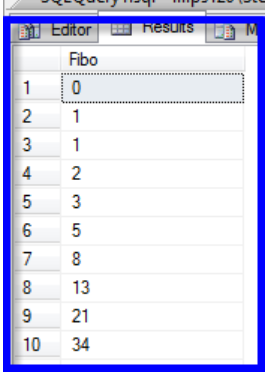# How to generate Fibonacci Series in SQL

Continuing my last article, I will discuss one more problem based on the RECURSIVE CTE.

This time I will cover the easy problem that you might have solved during your initial days of programming.

In this article, we learn to generate the Fibonacci Series in SQL.

# How to generate Fibonacci Series

I know most of us have solved this problem many times in other programming languages but not in SQL.

During our Initial programming days, this problem is taught to us to understand the concept of Recursion.

So we will also use the same Recursion concept to solve this problem.

Once again, the CTE starts with WITH RECURSIVE, followed by its name and the query definition. This time, I’ll use the anchor member of the recursive query to create some data. The columns are First_number and Second_number. This is the point I want the recursion to start from (the same way it was in the previous example, with level = 1).

Then comes the UNION ALL and the recursive member. This part of the query will increase the Second_number column by the sum of First_number and Second_number with every recursion, and the First_number column will be updated with the previous value of the Second_number column. The recursion will be performed in order to stop the recursion I will add any arbitrary condition (i.e. until it reaches the condition WHERE Second_number < 1000000000).

After that comes the simple SELECT statement that will return the column from the CTE. And here’s the result:-## Final Query:-

WITH RECURSIVE (First_number, Second_number) AS
(
SELECT 0, 1

UNION ALL

SELECT Second_number, First_number+ Second_number

FROM Fibonacci

WHERE  Second_number< 1000000000
)

SELECT Second_number AS Fibo
FROM Fibonacci
OPTION (MAXRECURSION 0);

Thanks for reading. I hope you enjoyed this Problem - it was fun for me to create!. You can also try a similar kind of problem

i.e. Print the Factorial of a number in SQL.